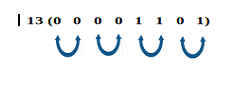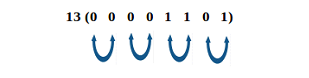# C++ program to swap adjacent bits

Swapping of adjacent bits in C++: Here, we are going to learn how to swap adjacent bits of a number?
Submitted by Saksham Bhayana, on December 12, 2018

Problem statement: C++ program to swap all odd bits with even bits (swap adjacent bits). Every even positiC++ program to swap all odd bits with even bits (swap adjacent bits). Every even position bit is swapped with an adjacent bit on the right side and every odd position bit is swapped with adjacent on the left side. For instance, 13(00001101) should be converted to 14(00001110) .on bit is swapped with an adjacent bit on the right side and every odd position bit is swapped with adjacent on the left side. For instance, 13(00001101) should be converted to 14(00001110).

Input format: The first line of input contains an integer T denoting the number of test cases. Then T test cases follow. The first line of each test case contains an unsigned integer N.

Output format: Corresponding to each test case, print in a new line, the converted number.

Constraints:

```    1 ≤ T ≤ 100
1 ≤ N ≤ 100
```

Example:

```    Input:
2
13
2

Output:
Original number is : 13
Converted number is : 14
Original number is : 2
Converted number is : 1
```

Explanation:

If the original number is 13 (00001101), then bits at odd and even position are swapped in following manner.After swapping, the bits are:Algorithm:

1. Create a mask to obtain the 0xAAAAAAAA to extract bits.
2. Obtain the odd bits and shift these to even positions.
3. Either create mask2 (0x55555555) for even bits or right shift the mask to extract it. Obtain the even bits and shift these to odd positions.
4. Combine the shifted values of odd and even bits to obtain the converted number.

Program:

```#include <iostream>
using namespace std;

unsigned int swap_odd_even(int num){
//A in hexadecimal is equal to 10 in decimal
//and 1010 in binary

//right shift for even bits
//can also use 0x55555555 as mask for even bits

return (oddbits>>1) | (evenbits<<1);
}

int main()
{
int T;  //testcases
cout<<"Enter total number of elements (test cases): ";
cin>>T;

//variable to store the number
unsigned int N;

for(int i=0;i<T;i++)
{
cout<<"Enter number: ";
cin>>N;
cout<<"Original number is : "<<N<<endl;
cout<<"Converted number is :"<<swap_odd_even(N)<<endl;
}

return 0;
}
```

Output

```Enter total number of elements (test cases): 2
Enter number: 13
Original number is : 13
Converted number is :14
Enter number: 2
Original number is : 2
Converted number is :1
```